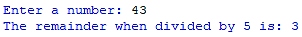## Calculating Remainders

The modulo operator - the percentage symbol % - will work out the remainder left over when one value is divided by another.==In the first example above 30 ÷ 6 = 5, which is a whole number, so there is no remainder.

In the second example 30 ÷ 7 = 4 remainder 2; so the remainder is output.

You can use modulo with variables too:=1. Ask the user to input a whole number.

2. Use the modulo operator to check if there is a remainder when the user's number is divided by 5.

3. Print the remainder.

Example solution:## Odd or Even?

The example below uses selection; which you won't have practised yet if you've been going through these pages in order.

To check if a number is odd or even you can see if it leaves a remainder when divided by 2:=Copy the example above for odd/even and edit the program to solve this problem:

A rollercoaster only lets people on in groups of 4.

1. Ask the user to input a whole number for how many people in their group.

2. Check if that number is directly divisible by 4. If it is then print “Perfect groups of four!”

3. Else print “You will be split up”.

Example solution:Embedded Python Editor